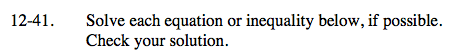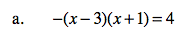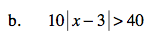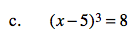### Home > AC > Chapter 12 > Lesson 12.2.1 > Problem12-41

12-41.Use a generic rectangle to multiply the factors together then distribute the negative and solve.

Set the equation equal to 0. Then use a generic rectangle to factor and solve for x using the Zero Product Property.Solve for the absolute value then make two equations to remove the absolute value.

x − 3 = 4 or x − 3 = −4
x = 7 or x = −1

Test numbers to determine which region is the solution.What number cubed equals 8?